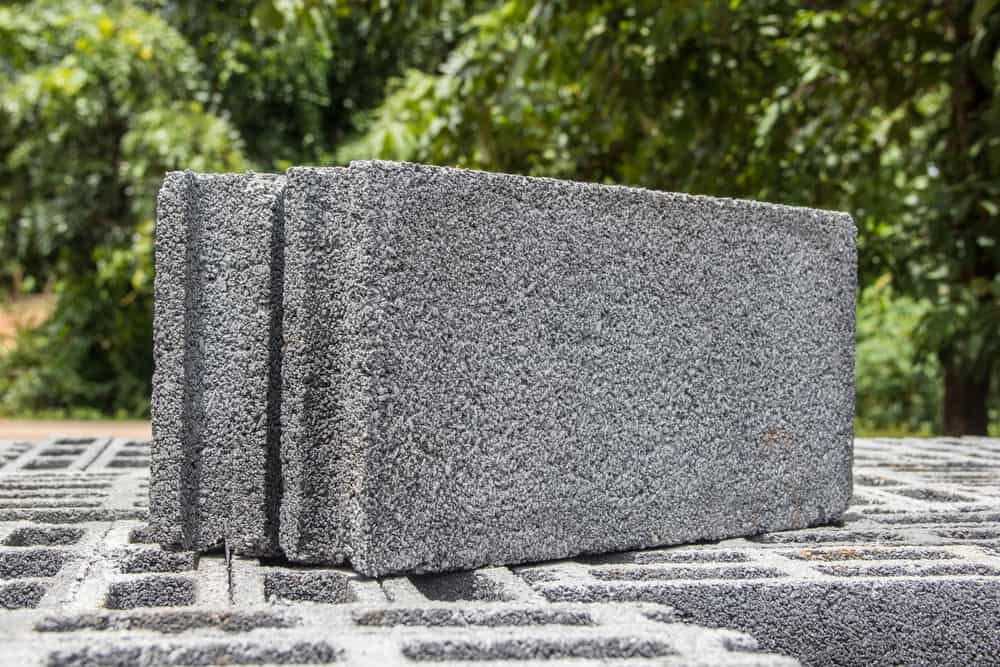Tags: Categories: Home Improvement

# Cinder Block Wall Cost Calculator

Do you need to figure out how many cinder blocks you need for your wall or other building project? Use this easy-to-use cinder block cost calculator that will tell you how many cinder blocks you need and approximately how much it will cost.Please note that the cost calculation does not include labor or mortar.  The approximate calculated cost only pertains to the cost of cinder blocks.

## How to use the following cinder block cost calculator:

Step 1: Determine the height of your wall or project in inches.  Input that figure in the first box.

Step 2: Determine the lenght of you cinder block project in inches.  Input that figure in the second box.

Once both steps complete, the calculator will display how many standard size cinder blocks you will need.  By standard size, we mean 8″ (H) x 8″ (D) x 16″ (W).  This dimension typically provides for mortar.

The final calculation is an approximate cost of your cinder blocks based on the cost from Home Depot (approximately \$2.50 per cinder block).

Related: Cinder block dimensions chart